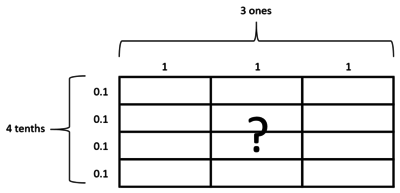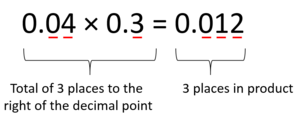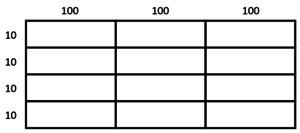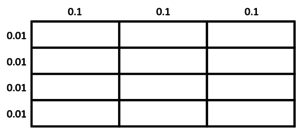# 12 Rectangular Units

Alignments to Content Standards: 6.NS.B.3

Here is a large rectangle made up of 12 smaller rectangles.This rectangle could represent the equation $0.4 \times 3 = ?$ if we label as shown below.The rectangle can also represent the following multiplication equations. For each one, draw a new rectangle and label the side lengths so it represents the multiplication equation. What is the area of a small rectangle? What is the product of the equation?

1. $40\times300=?$
2. $0.04\times0.3=?$

## IM Commentary

The purpose of this task is for students to notice how the decimal point behaves when numbers in different place-value places (for example, 0.04 and 0.3) are multiplied, and might be preceded by the task 2 Units Wide and 6 Units Long. It leverages students' understanding from grade 5 about patterns in the number of zeros of a product when multiplying by powers of 10 and patterns in the placement of a decimal point when a decimal is multiplied by a power of 10 [5.NBT.A.2]. Part (a) is a problem students should already be able to do as a result of their work in 5.NBT.B.7. With this task, we make sure that work is attached to a visual to set students up to understand multiplying by any number of decimals.

Students can quickly generate the grid diagrams if they are provided with blank graph paper. It might help for a teacher to quickly demonstrate creating a grid to draw on and thinking out loud about the sample product $0.4\times3$.

In follow-up discussion, a teacher should make sure everyone notices how the number of places work out, for example:Students may harbor some misconceptions or just poorly-understood procedures before beginning work on this task. For example, blindly counting decimal places in the product might lead a student to decide that $0.02 \times 0.5 = 0.001$ instead of $0.01$ (which comes from $0.010$). To promote understanding, we suggest two approaches. First, in class discussion of solutions, route each problem back to the area of rectangles. In the diagram of $0.04 \times 0.3$, you can see that the area, which is the same value as this product, consists of twelve rectangles each with an area of $0.001$, and twelve of these make $0.012$. Secondly, this reasoning could be written down explicitly using the associative and commutative properties of multiplication:

$$0.04\times0.3$$

$$(4\times0.01)\times(3\times0.1)$$

$$(4\times3)\times(0.01\times0.1)$$

$$12\times0.001$$

$$0.012$$

## Solution

1. $40\times300$The area of a small rectangle is $100\times10=1000$. Since there are 12 of these small rectangles, the product is $12,000$.
2. $0.04\times0.3$The area of a small rectangle is $0.1\times0.01=0.001$. Since there are 12 of these small rectangles, the product is $0.012$.